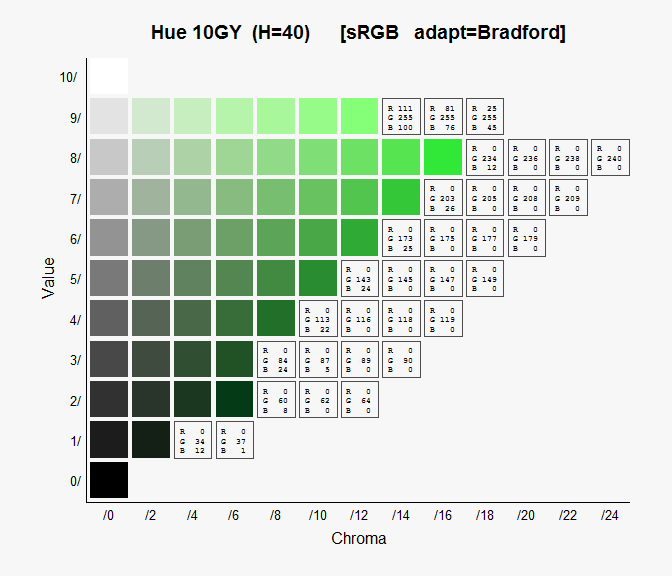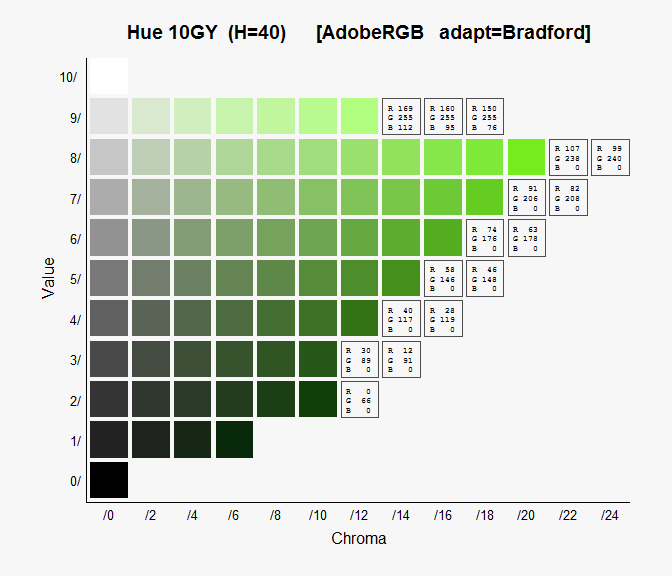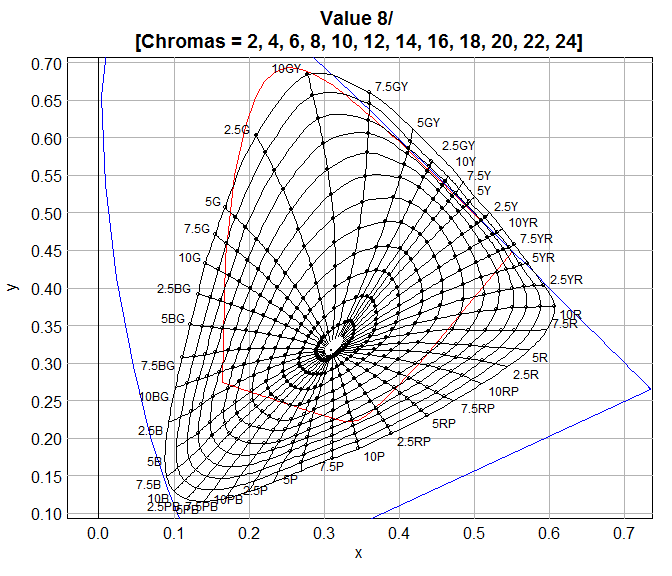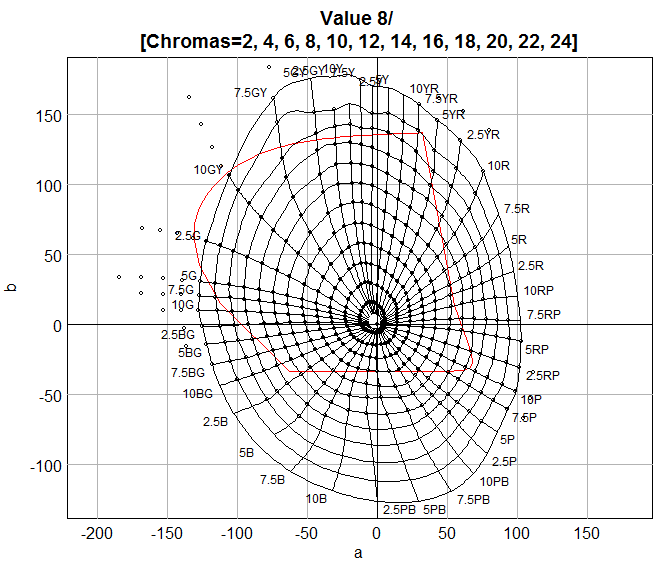# munsellinterpol User Guide

#### 2020-02-01

munsellinterpol is an R package that transforms from Munsell HVC to CIE xyY, and back again. Our package was inspired by Paul Centore’s An Open-Source Inversion Algorithm for the Munsell Renotation .

The goals of this package are:

1. in the forward conversion HVC $$\to$$ xyY, if HVC is in the published tables ( and ) then the output xyY must match the tables
2. accurate round-trips HVC $$\to$$ xyY $$\to$$ HVC, and xyY $$\to$$ HVC$$\to$$ xyY
3. conversion for all colors within the MacAdam limits, including Munsell Values < 1
4. both conversions HVC $$\leftrightarrow$$ xyY are class $$C^1$$ (continuously differentiable), except at the neutrals where $$C^0$$ (continuous) is acceptable

Goals 1, 2, and 3 have been met. This means that extensive tests were passed, with many color samples. There were initial problems with very dark samples where V $$<$$ 1, so it is possible there could be some other dark samples that were missed, and where goal 3 fails.

In attempting to meet goal 4, the forward map HVC $$\to$$ xyY uses Catmull-Rom spline interpolation in V (by default). But because of a change in Value spacing, the function HVC $$\to$$ xyY is not $$C^1$$ on the plane V=1. When V $$\ge$$ 1 the Value spacing is 1, but when V $$\le$$ 1 the Value spacing is 0.2. So goal 4 has not been met. For H and C bicubic interpolation is used, again with Catmull-Rom splines; see  and . There are options to use simpler linear interpolation for V, and bilinear interpolation for H and C. These are useful for comparison with other algorithms, such as . Of course, to meet goal 2 the options for forward and inverse maps must be the same.

Note that goal 1 does not include points from all.dat. This goal is actually met for V $$\ge$$ 1, but for V $$<$$ 1 we had to ignore the points from all.dat and re-extrapolate to make inversion work reliably. In general, in this package the xy for these non-real points is further from white than the corresponding points in all.dat. For the meaning of all.dat see Appendix A.

The package dependencies are:

• geometry  - for building the convex hull of the object colors of Illuminant C
• rootSolve  - for inverting the forward conversion HVC $$\to$$ xyY

The package microbenchmark  is suggested, for its high-precision timer.

Similar packages: munsell  also does HVC $$\leftrightarrow$$ sRGB conversion, but only for discrete HVCs from the Munsell Book; there is no interpolation.

We assume that the reader is familiar with Munsell Hue, Value, and Chroma, which we abbreviate by HVC. A good introduction is . All 3 are stored as floating-point numbers: H is in the interval (0,100], V is in [0,10], and C is non-negative with upper limit a complex function of H and V. These are cylindrical coordinates on the irregular Munsell object color solid. We assume that the reader is familiar with the Munsell character string notation H V/C for chromatic colors, and N V/ for achromatics (neutrals).

We assume that the reader is familiar with sRGB; a good reference is .

We assume that the reader is familiar with the CIE spaces xyY, XYZ, and Lab. Y is the luminance factor and in this domain is considered to be a percentage in the interval [0,100]. A perfectly black surface has Y=0, and a perfectly reflecting diffuser has Y=100. An excellent reference is .

There are, and have been, many other software programs that do these conversions. The earliest one we know of is  (1960), which ran on an IBM 704. See  for a discussion of many other software programs and algorithms.

# Basics

The forward conversion HVC $$\to$$ xyY is as simple as:

MunsellToxyY( '4.2RP 5.5/8' )
##   SAMPLE_NAME HVC.H HVC.V HVC.C      xyY.x      xyY.y      xyY.Y
## 1 4.2RP 5.5/8  94.2   5.5   8.0  0.3624688  0.2733678 23.9680791

The return value is a data.frame. The first column SAMPLE_NAME is the input Munsell notation. The second column HVC is its numerical version. And the 3rd column is the output xyY. If the input is a character vector of length N, then the data.frame has N rows. The input can also be a numeric matrix HVC with N rows. There are many options for advanced users, see the man page for details.

And here is an example of the reverse conversion xyY $$\to$$ HVC:

xyY = MunsellToxyY( '4.2RP 5.5/8' )$xyY xyYtoMunsell( xyY ) ## xyY.x xyY.y xyY.Y HVC.H HVC.V HVC.C SAMPLE_NAME ## 4.2RP 5.5/8 0.3624688 0.2733678 23.9680791 94.2 5.5 8.0 4.2RP 5.5/8 And so the round-trip has returned us to 4.2RP 5.5/8. In general, the round-trip is accurate to about 2 decimal places; the worst case is the Hue at near neutrals. Note that the return value is also a data.frame, but now SAMPLE_NAME comes at the end. Other color spaces available are: sRGB, XYZ, Lab, Luv, and Colorlab. All the conversion functions accept multiple “samples”. Some of the functions return a data.frame and some return a plain matrix. See the Reference Guide for details. # Plotting The most obvious thing to plot is a simulation of a page from the Munsell Book of Color: par( omi=c(0,0,0,0), mai=c(0.6,0.7,0.4,0.2) ) plotPatchesH( "10GY", back='#f7f7f7' )This is the forward conversion HVC $$\to$$ xyY $$\to$$ xyY $$\to$$ sRGB. There is no interpolation here, unless the Hue is not a multiple of 2.5, which is allowed. For each Value, the Chroma is extended up to the MacAdam limit, and this determines the limit of the Chroma axis. If the sRGB for a patch is outside the cube (before clamping), it is not drawn and the sRGB values are printed instead. This makes it easy to see why the patch is outside the sRGB gamut. The chromatic adaption method, the background color, and the main title can be controlled. Note that the plot is titled with sRGB, and this plot is best viewed on a display calibrated for sRGB. Adobe RGB space is also available. par( omi=c(0,0,0,0), mai=c(0.6,0.7,0.4,0.2) ) plotPatchesH( "10GY", space='AdobeRGB', back='#f7f7f7' )Of course, this one is best viewed on a display calibrated for Adobe RGB. Note that the gamut is quite a bit larger in this figure. Users can add custom RGB spaces too. In the Munsell arena, another standard plot is the curves of constant Hue and Chroma. par( omi=c(0,0,0,0), mai=c(0.6,0.7,0.6,0.2) ) plotLociHC( value=8 )The black-filled circles are from real.dat (see Appendix A), and the open circles are extrapolated from the “real” ones. The blue curve is the CIE inverted-U, and the red curve is the MacAdam limit for the given Value=8. One can also plot in the a,b plane. par( omi=c(0,0,0,0), mai=c(0.6,0.7,0.6,0.2) ) plotLociHC( value=8, coords='ab' )The interpolation takes place in x,y and is then mapped to a,b. For similar plots, see the Loci of Contant Hue and Chroma vignette. # ISCC-NBS Color Names The ISCC-NBS System is a partition of Munsell Color Solid into 267 color blocks, see . Each block has an ISCC-NBS Name; one of the goals of these names is that they should be “simple enough to be understood by the average person on the street”. Each block is a disjoint union of elementary blocks (of which there are 932) where an elementary block is defined by its minimum and maximum limits in Hue, Value, and Chroma. The peripheral blocks (of which there are 120) have arbitrary large chroma (maximum Chroma is $$\infty$$). Each block has an associated centroid color, see , which is in the interior of the block (even though some blocks are non-convex). Given a query point HVC, the function ColorBlockFromMunsell() searches for the one elementary block that contains that point. Since 2000, the Pantone Color Institute has declared a “Color of the Year”, see . And they publish the sRGB coordinates of the that color. We thought it would be interesting to compare the Pantone colors with the corresponding ISCC-NBS Names and centroids. Read the data for all colors since 2010. path = system.file( 'extdata/PantoneCoY.txt', package='munsellinterpol' ) pantone = read.table( path, header=TRUE, sep='\t', strings=FALSE ) pantone ## Year Name Code R G B ## 1 2010 Turquoise 15-5519 69 181 170 ## 2 2011 Honeysuckle 18-2120 217 79 112 ## 3 2012 Tangerine Tango 17-1463 221 65 36 ## 4 2013 Emerald 17-5641 0 148 115 ## 5 2014 Radiant Orchid 18-3224 177 99 163 ## 6 2015 Marsala 18-1438 149 82 81 ## 7 2016 Rose Quartz 13-1520 247 202 201 ## 8 2016 Serenity 15-3913 146 168 209 ## 9 2017 Greenery 15-0343 136 176 75 ## 10 2018 Ultra Violet 18-3838 95 75 139 ## 11 2019 Living Coral 16-1546 255 111 97 Add the block names and centroids. pantone$Year = NULL ;   pantone$Code = NULL RGB = as.matrix( pantone[ , c('R','G','B') ] ) HVC = RGBtoMunsell( RGB, space='sRGB' ) pantone$Munsell = MunsellNameFromHVC( HVC )
block = ColorBlockFromMunsell( HVC )
pantone[[ "ISCC-NBS Name" ]] = block$Name pantone[[ "ISCC-NBS Centroid" ]] = block$Centroid
pantone
##               Name   R   G   B       Munsell         ISCC-NBS Name ISCC-NBS Centroid
## 1        Turquoise  69 181 170 4.9BG 6.6/6.8    light bluish green     4.5BG 6.5/5.0
## 2      Honeysuckle 217  79 112  0.16R 5.3/13   strong purplish red    7.0RP 4.5/11.9
## 3  Tangerine Tango 221  65  36     8.5R 5/15  vivid reddish orange     9.5R 5.5/15.5
## 4          Emerald   0 148 115  7.7G 5.3/7.7          strong green      6.0G 4.5/9.1
## 5   Radiant Orchid 177  99 163   9.7P 5.2/11 strong reddish purple    1.0RP 4.5/11.1
## 6          Marsala 149  82  81  4.8R 4.2/6.1           grayish red      4.6R 4.5/4.7
## 7      Rose Quartz 247 202 201    2.9R 8.5/4            light pink      2.5R 8.6/5.2
## 8         Serenity 146 168 209 5.9PB 6.8/6.1   light purplish blue     7.5PB 6.0/6.8
## 9         Greenery 136 176  75 6.4GY 6.6/8.1   strong yellow green     5.0GY 6.0/9.1
## 10    Ultra Violet  95  75 139 0.87P 3.6/8.6       moderate violet      1.0P 3.5/7.2
## 11    Living Coral 255 111  97   6.6R 6.4/13   deep yellowish pink     5.6R 6.0/12.4

Note that Emerald is unfortunately outside the sRGB gamut. Now compare the original color, and the centroid approximation to it.

color.pant = rgb( RGB, max=255 )
color.cent = rgb( MunsellToRGB( block$Centroid, space='sRGB' )$RGB, max=255 )
tbl = data.frame( row.names=1:nrow(pantone) )
tbl[[ "Pantone Name" ]]  = pantone$Name tbl[[ "Pantone Color" ]] = '' ; tbl[[ "Centroid Color" ]] = '' tbl[[ "ISCC-NBS Name" ]] = block$Name
library( flextable )
myrt <- regulartable( tbl )
myrt <- align( myrt, j=4, align='left', part='all' )
myrt <- align( myrt, j=2:3, align='center', part='all' )
myrt <- height( myrt, height=1 )
myrt <- width( myrt, j=c(1,4), width=2 ) ; myrt <- width( myrt, j=2:3, width=2.5 )
myrt <- fontsize( myrt, size=14, part='all' ) ; myrt <- fontsize( myrt, size=16, part='header' )
for( i in 1:nrow(tbl) )
{ myrt <- bg(myrt, i=i, j=2, bg=color.pant[i]) ; myrt <- bg(myrt, i=i, j=3, bg=color.cent[i]) }
myrt

 Pantone Name Pantone Color Centroid Color ISCC-NBS Name Turquoise light bluish green Honeysuckle strong purplish red Tangerine Tango vivid reddish orange Emerald strong green Radiant Orchid strong reddish purple Marsala grayish red Rose Quartz light pink Serenity light purplish blue Greenery strong yellow green Ultra Violet moderate violet Living Coral deep yellowish pink

This table is best viewed on a display calibrated for sRGB. The color match is poor - clearly the ISCC-NBS designation is coarser than the Pantone designation. In fact, in the 6 levels of color designation (from coarsest to finest) in , ISCC-NBS is Level 3 and Pantone would most likely be Level 4.

# Possible Future Work

Here are a few possible improvements and additions.

The conversions are already pretty fast. On my PC with 3GHz Intel Core Duo, the forward conversion HVC $$\to$$ xyY takes about 1 msec/sample, and the reverse xyY $$\to$$ HVC takes about 8 msec/sample. This assumes a large number of samples per function call. The next obvious speed-up is to move the interpolation code for the forward conversion from R to compiled C. It would have the added benefit that the 2x2 Jacobian could also be computed during the inversion xyY $$\to$$ HVC, which would reduce the number of function evaluations per Newton-Raphson iteration from 3 to 1.

For V $$<$$ 1, it might be possible to restore the extrapolated points from all.dat. But it will probably take better 3D visualization to assist in viewing the xy irregularities in these very dark colors.